0

# python 文件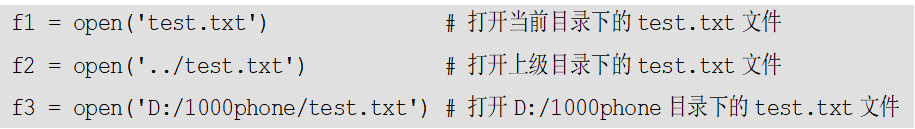``````open( file ,[,mode = 'r' [,...] ])
``````
``````file
``````

``````mode
``````

``````with open('freedom.txt',encoding="utf-8") as f:
``````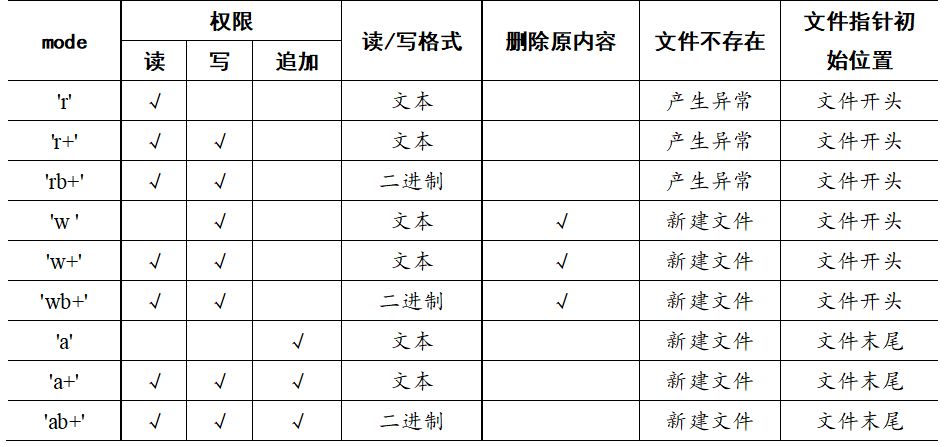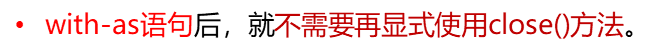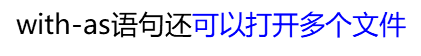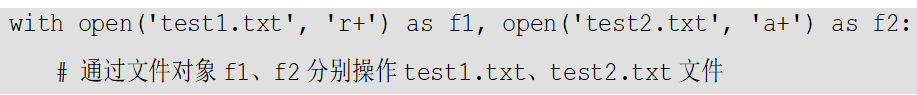``````withopen('freedom.txt',encoding="utf-8")as f:whileTrue:
``````

Python对文件的操作中，

``````read
``````

``````readline( )
``````

in 关键字``````文件名.write（）
``````
``````f2 =open('dic.txt','w')
di={}for k,v in di.items():
s=k+":"+str(v)+'\n'
f2.write(s)
``````

``````tell()
``````

Python标准库

``````os.path
``````

``````isdir()
``````

Python标准库

``````os.path
``````

``````isfile()
``````

``````os.remove( filename )
``````

#filename: 要删除的文件名

``````filename
``````

``````filename
``````

``````exists()
``````

Python标准库os.path中用来判断指定文件或目录是否存在的方法

``````flush()
``````

### “python 文件”的评论:

##### 关于作者##### overfit同步小助手